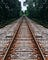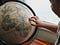Photo by Bruno Wolff on Unsplash

# Distance as MetricsPhoto by Tom Barrett on Unsplash

# Two Dimensions

## Manhattan Distance

`def manhattan(a, b):    manhattan_distance = 0    for i in [0, 1]:        manhattan_distance += abs(a[i] - b[i])    return manhattan_distancea = (4, 2)b = (0, 1)manhattan(a,b)5`

## Euclidean Distance

`from math import sqrt def euclidean(a, b):    euclidean_distance = 0    for i in [0, 1]:        euclidean_distance += (a[i] - b[i])**2    return sqrt(euclidean_distance)a = (4, 2)b = (0, 1)euclidean(a,b)4.123105625617661`

# Three Dimensions or More

## Manhattan Distance

`def manhattanXD(a, b, dimensions=2):    manhattan_XD = 0    for i in range(dimensions):        manhattan_XD += abs(a[i] - b[i])    return manhattan_XDa = (4, 2, 2)b = (0, 0, 1)manhattanXD(a, b, 3)7`

## Euclidean Distance

`from math import sqrt def euclideanXD(a, b, dimensions=2):    euclidean_XD = 0    for i in range(dimensions):        euclidean_XD += (a[i] - b[i]) ** 2    return sqrt(euclidean_XD)a = (4, 2, 2)b = (0, 0, 1)euclideanXD(a, b, 3)4.58257569495584`

## Minkowski Distance

`import numpy as npdef minkowski(a, b, dimensions=2, power=1):    minkowski_XD = 0    for i in range(dimensions):        minkowski_XD += abs(a[i] - b[i]) ** power    return np.power(minkowski_XD, 1/power)a = (4, 2, 2)b = (0, 0, 1)minkowski(a, b, dimension=3, power=3)4.179339196381232`

## Cosine Distance

`a = (4, 2, 2)b = (0, 0, 1)def cosine_distance(a, b):    normalised_a = np.linalg.norm(a)    normalised_b = np.linalg.norm(b)    dot_product = np.dot(a, b)    return 1 - (dot_product/(normalised_a * normalised_b))cosine_distance(a, b)0.5917517095361369`

# Haversine DistancePhoto by Amy Humphries on Unsplash
`def haversine_distance(lat1, lon1, lat2, lon2):    p1 = np.radians(lat1)    p2 = np.radians(lat2)    l1 = np.radians(lon1)    l2 = np.radians(lon2)    hav = np.sin((p2 - p1) / 2) ** 2 + np.cos(p1) * np.cos(p2) * np.sin((l2 - l1) / 2) ** 2    dist = 2 * 6371 * np.arcsin(np.sqrt(hav))    return np.round(dist, 2)london = (51.509865, -0.118092)paris = (48.864716, 2.349014)haversine_distance(*london, *paris)342.54`

Data Scientist